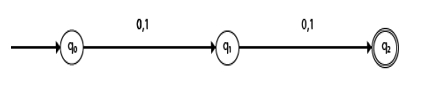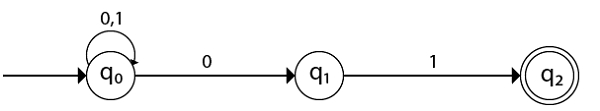# Design NFA with Σ = {0, 1} and accept all string of length at least 2.

Non-deterministic finite automata also have five states which are same as DFA, but with different transition function, as shown follows −

δ: Q X Σ -> 2Q

Non-deterministic finite automata is defined as a 5 tuple,

M=(Q, Σ, δ,q0,F)

Where,

• Q: Finite set of states
• Σ: Finite set of the input symbol
• q0: Initial state
• F: Final state
• δ: Transition function: Q X Σ -> 2Q

## Problem

Design a transition diagram and table for the given language that accepts all strings of length at least 2.

## Solution

Before proceeding to the solution, let’s understand what do you mean by length of string and how to find the length of any string.

The length of a string means it is the number of symbols in the string or word. It is denoted by |w|.

For Example: w=01011001 from binary alphabet Σ={0,1}

|w| = 8

In a given problem, the strings should have length of at least 2. That is, the length should be 2 or more, but not less than 2 over the alphabet Σ = {0, 1}.

The language L= { 00,01,10,11,001,110,101,111,000,1101,0010,…….}

## Transition Diagram

The transition diagram of NFA for the given language is −## Transition table

The transition table is as follows −

Present stateNext state for input 0Next state for input 1
->q0q1q1
q1q2q2
*q2εε

## Explanation

• Step 1 − q0 is an initial state on input ‘0’ it goes to state q1 and on input ‘1’ goes to state q1.
• Step 2 − q1 is an intermediate state on input ‘0’ and ‘1’ goes to state q2.
• Step 3 − q2 is the final state, it contains epsilon transitions.

## Example 2

Construct an NFA with Σ = {0, 1} which accepts all string ending with 01.

In a given problem, the language accepts all strings ending with 01.

The language L= { 01,101,1101,001,11001,0101,11101,0001,1001,00101,…….}

## Transition diagram

The transition diagram is as follows −## Transition Table

The transition table is as follows −

Present stateNext state for input 0Next state for input 1
->q0q0,q1q0
q1-q2
*q2--

## Explanation

• Step 1 − q0 is an initial state on input ‘0’ ii goes to multiple states q0 and q1 and ‘1’ it goes to state q0 itself.
• Step 2 − q1 is an intermediate state on input ‘1’ goes to state q2.
• Step 3 − q2 is the final state, it contains epsilon transitions.